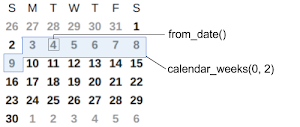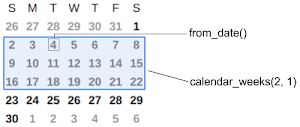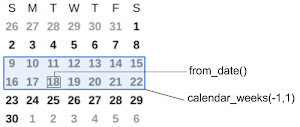# calendar_weeks

Returns the current week, and can count forward or backwards a specified number of additional weeks. Can only be used as the second `date` parameter in for_date_range(date, date). All counting is relative to the first `date` parameter in `for_date_range(date, date)`.

## Syntax

`calendar_weeks(numberOfWeeks, [startDay])`

### Parameters

• numberOfWeeks:  A value of zero returns the entire week that the first `date` parameter is in. A postive integer specifies an additional number of full weeks to count forward. A negative integer specifies a number of full weeks to count backwards in addition to the current full week.
• startDay: Optional. An integer (1 through 7) that specifies which day you consider to be the first day of the week:
• `1` = Sunday
• `2` = Monday
• `3` = Tuesday
• `4` = Wednesday
• `5` = Thursday
• `6` = Friday
• `7` = Saturday

By default, Sunday is the starting day.

## Examples

• `Clicks.for_date_range(from_date(), calendar_weeks(0,2))`: Returns the number of clicks for the full Monday-to-Sunday week that contains from_date().

In the following example:
• The report's time range starts on the 4th, so `from_date()` returns the 4th.
• The second parameter specifies that weeks start on Monday.
• `calendar_weeks(0,2)` returns the number of clicks from the 3rd to the 9th (Monday to Sunday).• `Clicks.for_date_range(from_date(), calendar_weeks(2,1))`: Returns the number of clicks for the full week that contains from from_date() and ending two full weeks later.

In the following example:
• The report's time range starts on the 4th, so `from_date()` returns the 4th.
• `calendar_weeks(2,1)` returns the full week that contains the 4th, and then finds the next two Sunday-to-Saturday weeks.
• The entire function returns the number of clicks from the 2nd to the 22nd.• `Clicks.for_date_range(from_date(), calendar_weeks(-1,1))`: If `from_date()` returns the 18th, then the overall function returns the number of clicks from the 9th (which is the first full week that precedes the 18th) to the 22nd (which is the full week that includes the 18th).